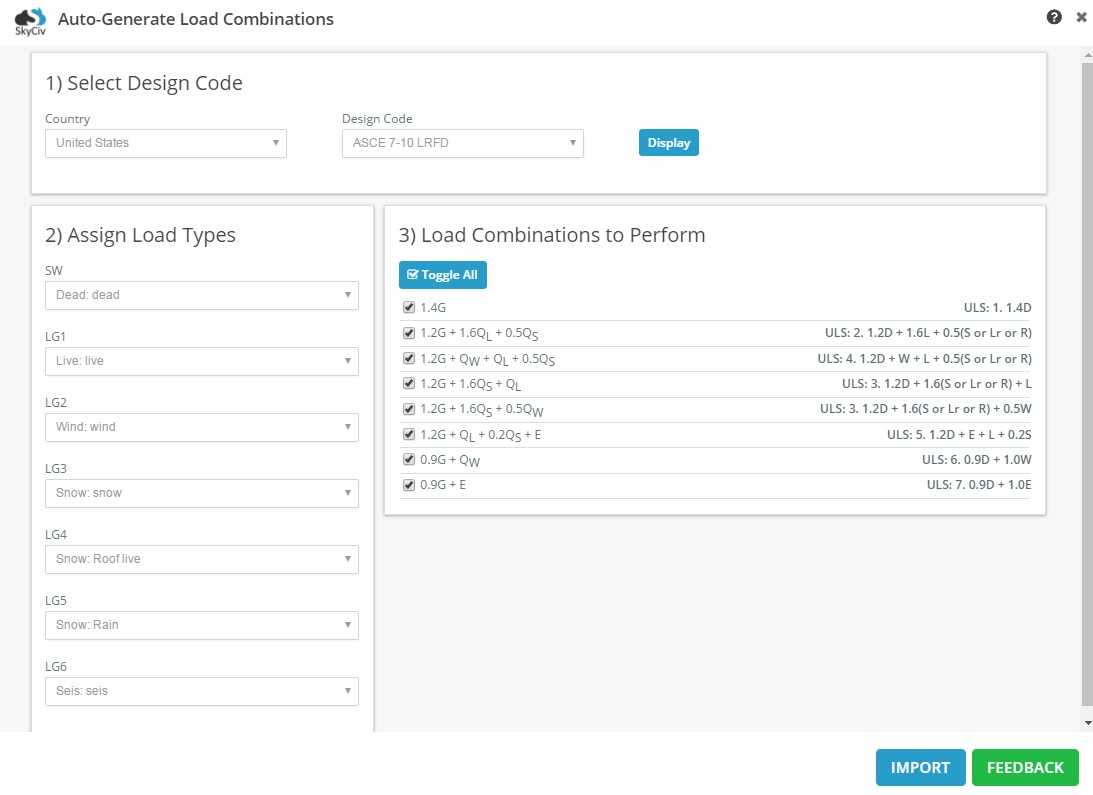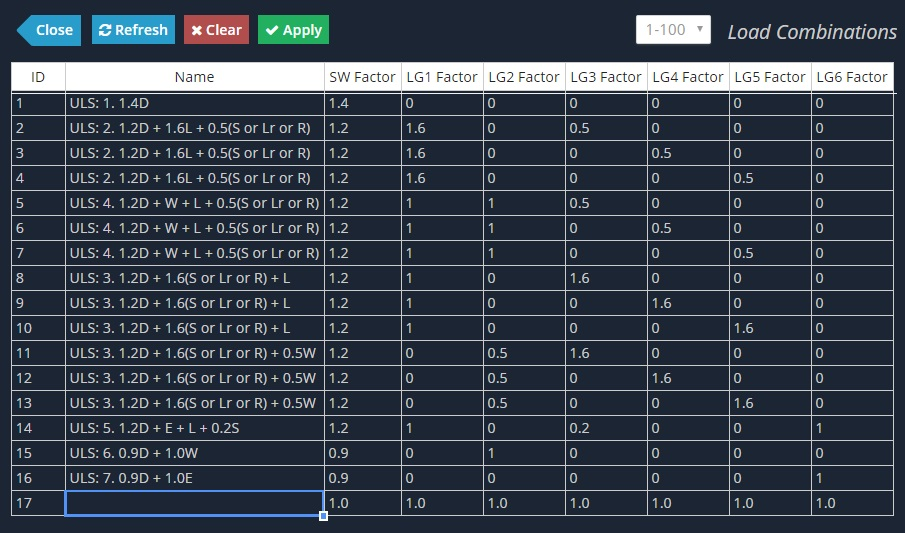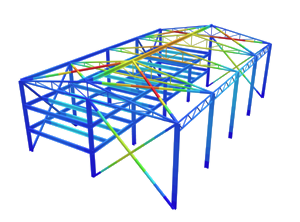SkyCiv Documentation

Your guide to SkyCiv software - tutorials, how-to guides and technical articles

1. Home
2. TechNotes
4. ASCE 7-10 LRFD Load Combinations

# ASCE 7-10 LRFD Load Combinations

## A guide to Load Combinations: ASCE 7-10 LRFD

In the United States, ASCE 7-10 is an key part of the building code. It focuses on the requirements for general structural design, as well as providing a means for determining loads (dead, live, soil, flood, snow, rain, ice, earthquake, wind) and their combinations.

Load and Resistance Factor Design (LRFD) involves seven basic load combination equations.

This article will focus on how SkyCiv’s auto generated load combinations feature meets the load combination equations as specified in ASCE 7-10 LRFD.

## 2.3.2 Basic Combinations

Design Code Equation Design Code Comment SkyCiv Equation SkyCiv Comment
1.4D Eq. 2.3.2.1 1.4G
1.2D + 1.6L + 0.5(Lrr or S or R) Eq. 2.3.2.2 1.2G + 1.6QL + 0.5QS The load type “Snow” is sub-categorized into “snow”, “roof live”, and “rain”. Selecting this load combination will automatically create 3 load combination equations due to the use of “or” in the equation specification.
1.2D + 1.6(Lrr or S or R) + (L or 0.5W) Eq. 2.3.2.3 1.2G + 1.6QS + QL
1.2G + 1.6QS + 0.5QW
Due to the presence of “or” in two places of this load combination, this generates 6 load equations. SkyCiv has separated this into two load combinations (for the L or 0.5W options) which generates 3 load equations each.
1.2D + 1.0W + L + 0.5(Lr or S or R) Eq. 2.3.2.4 1.2G + QW + QL + 0.5QS The load type “Snow” is sub-categorized into “snow”, “roof live”, and “rain”. Selecting this load combination will automatically create 3 load combination equations due to the use of “or” in the equation specification.
1.2D + 1.0E + L + 0.2S Eq. 2.3.2.5 1.2G + QL + 0.2QS + E
0.9D + 1.0W Eq. 2.3.2.6 0.9G + QW
0.9D + 1.0E Eq. 2.3.2.7 0.9G + E

## Example: All Load Types Assigned

Whilst not practical, 7 load groups have been created and assigned to all 7 load types as seen below. This is done to check the equations that are formed from the load combinationsThe results from the datasheet are shown below.